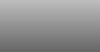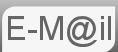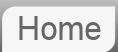# Answer to Riddle #85: Garden Path

85. A man has a rectangular garden, 55m ('B' in the diagram,) by 40m('A',) and he makes a diagonal path, 1m wide, exactly in the manner indicated in the diagram. What is the area of the path?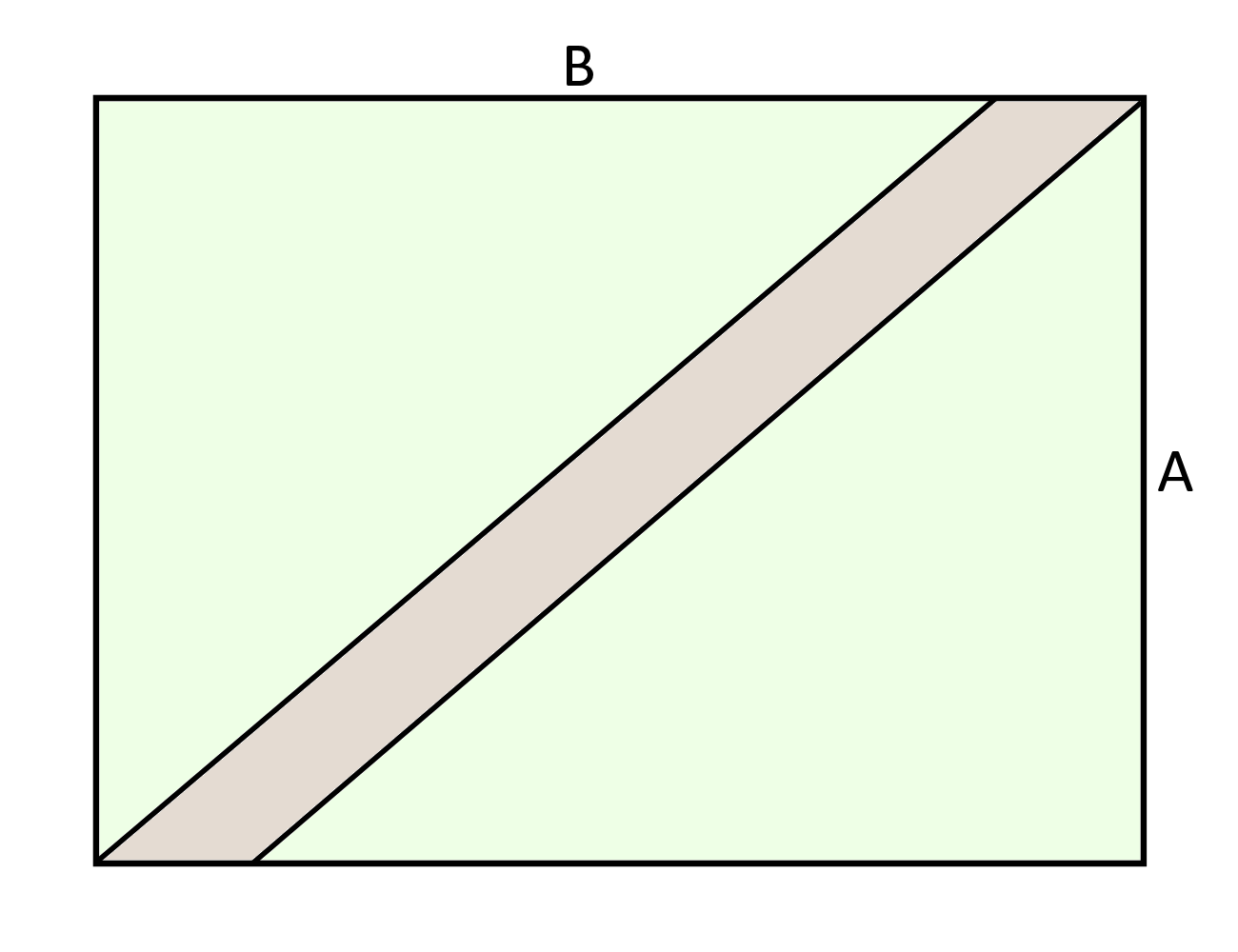At first this puzzle looks trivial, just simple geometry. It's not. It's tricky:

Lets label up a diagram so we know what we're talking about. Note the diagonal path, in the corners, does not meet in the way you might expect:

We are going to use two relationships to solve for the area of the path: Pythagerous on the triangle (A,B-z,L+y), and that the area of the path + the area of the grass is equal to the total area (A•B).

The area of the path is given by (L+y)•x, if that's not clear then it should be from the following diagram, where we imagine a triangle removed from the bottom left to the top right, showing that it forms a rectangle.

So the equation for the areas is as follows:And the equation using Pythagerous on the △(A,B-z,L+y):From here we can rearrange the first equation in terms of (L+y) and substitute it in to the second. We will ultimate form a quadratic equation for z which we will solve in the usual way, then solve solve for the area of the path by plugging 'z' into the equation where where we equated the areas:At this point it's honestly easier to just plug the numbers in, (it's particularly helpful that x=1,) and you should be able rearrange to a quadratic that looks like the following:Which solves to give z=1 2⁄3, which we can use in our very first equation. The first equation basically says the area of the path = total area (2200m2,) - the area of the grass. Which using our value of z=1 2⁄3 gives us and area for the path of 66 2⁄3m2

Puzzle comes from a book, 536 Puzzles by Martin Gardner

© Nigel Coldwell 2004 -  – The questions on this site may be reproduced without further permission, I do not claim copyright over them. The answers are mine and may not be reproduced without my expressed prior consent. Please inquire using the link at the top of the page. Secure version of this page.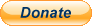PayPal
I always think it's arrogant to add a donate button, but it has been requested. If I help you get a job though, you could buy me a pint! - nigel